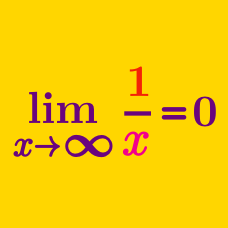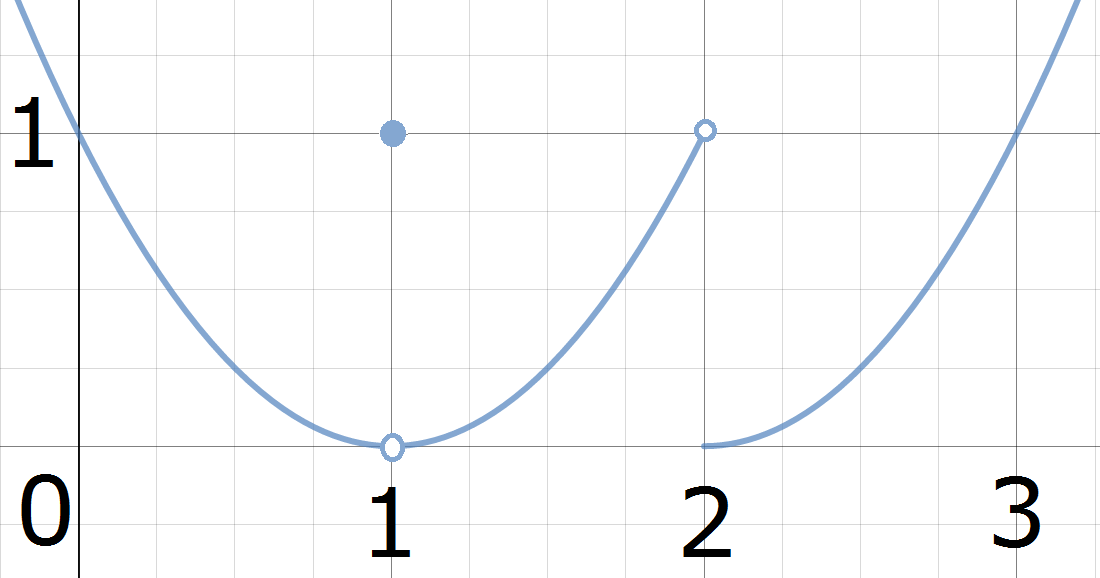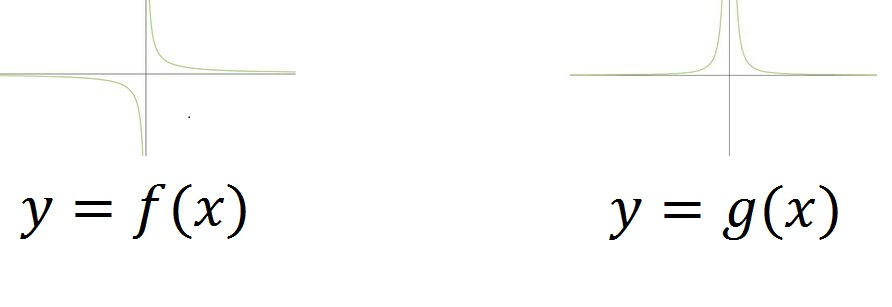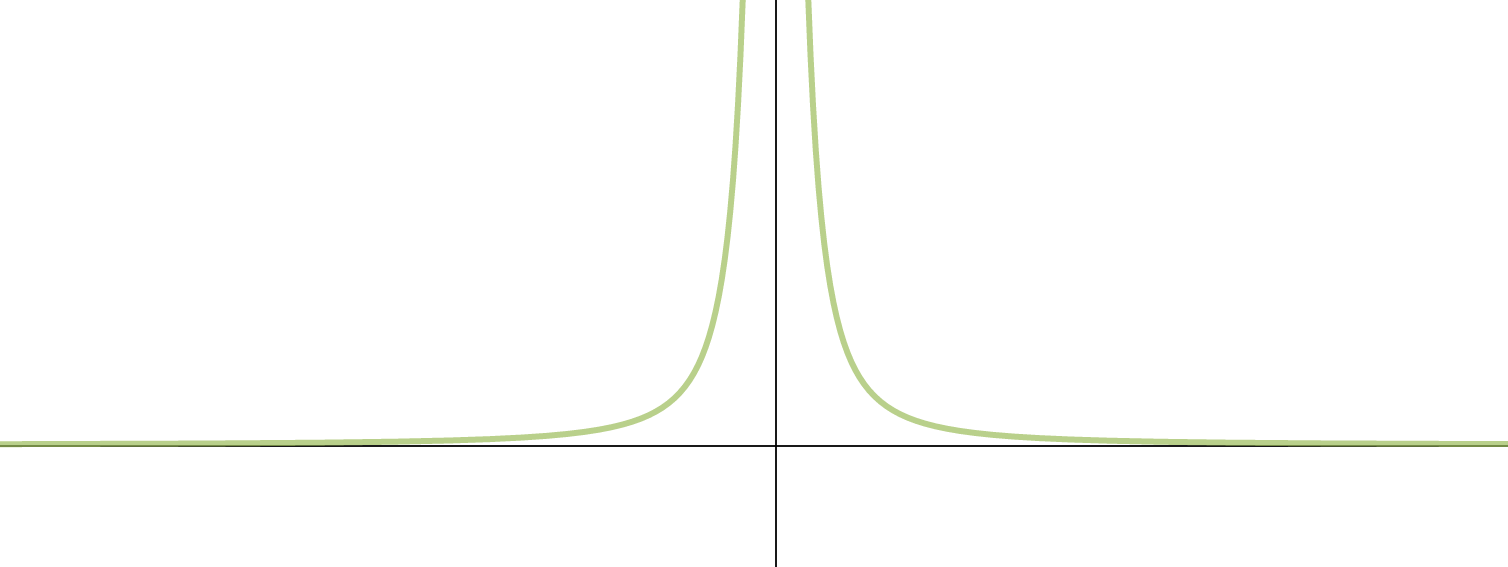Calculus

# When Limits Don't ExistThe graph of $y = f(x)$ is pictured above. For which values of $a$ in $\{0, 1, 2\}$ does $\lim_{x \to a} f(x)$ exist?Based on the graphs, which limit diverges to $\infty$ as $x \to 0?$$\lim_{x \to a} \frac{1}{x^2} = \text{ a (finite) number}$

For which choice of $a$ is the above statement true?

Which option is true of $A = \lim_{x \to \infty} \sin\left(x\right)?$

$A = \lim_{x \to 0} \frac{x}{x}, \,\,\,\,\,B = \lim_{x \to 0} \frac{|x|}{x}$

Which limit(s) exist(s)?

×